Next: Numerical simulation Up: Discreteness and its effect Previous: Steady state solutions.

# Resonant interaction in discrete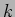-space

Importance of the-space discreteness for weakly nonlinear waves in finite basins were realized by Kartashova  and it was later discussed in a number of papers, [14,28,29,30]. Nonlinear wave interactions crucially depend on the sort of resonances the dispersion relation allows. The dispersion relation of the surface gravity waves is concave and hence it forbids three-wave interactions, so that the dominant process is four-wave. Resonant manifold is defined by resonant conditions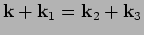,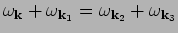or, substituting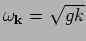,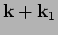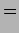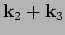(29)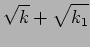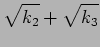(30)

The problem of finding exact resonances in discrete-space was first formulated by Kartashova  who gave a detailed classification of for some types of waves, e.g. Rossby waves. For the deep-water gravity waves, Kartashova only considered symmetric solutions, i.e. of the form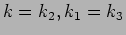(or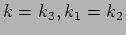). Interestingly, there appear to be also asymmetric solutions,

Solutions for collinear quartets and the tridents are easy to find by rewriting the resonant conditions (25) as polynomial equations (appropeiately re-arranging and taking squares of the equations) and using rational parametrisations of their solutions. This way we get the following family of the collinear quartets ,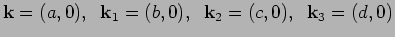with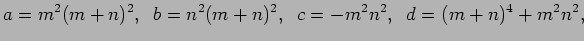where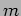and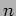are natural numbers, and tridents'',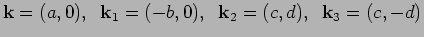with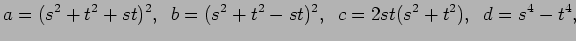where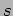and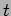are integers. Both of these classes can be easily extended by re-scaling, i.e. multiplying all four vectors by an integer. Even more solutions can be obtained via rotation by an angle with rational-valued cosine (this gives a new rational solution) and further re-scaling (to obtain integer solution out of the rational one).

There are other, rather rare, exact nontirvial resonances, for example one provided to us by Kartashova :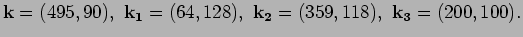The complete investigation of the exact resonance types and their respective roles is a facinating subject of future work).

Note that the interactions coefficient vanishes on the collinear quartets  and, therefore, these quartets do not contribute into the turbulence evolution. Secondly, there appears to be much more symmetric quartets than tridents, so the later are relatively unimportant for the nonlinear dynamics too.

Because of nonlinearity, the wave resonances have a finite width. Even though this width is small in weakly nonlinear systems, it may be sufficient for activating new mode interactions on the-space grid and thereby trigger the turbulent cascade through scales. Such quasi-resonances can be roughly modeled through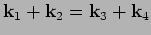,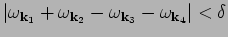, where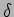describes the resonance broadening. Figure 1 shows quasi-resonant generations of modes on space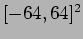, where initially (generation 1) only modes in the ring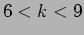were present (as in our numerical experiment). With broadening of the resonant manifold smaller than the critical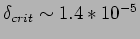, a finite number of modes outside the initial region get excited due to exact resonances (generation 2) but there are no quasi-resonances to carry energy to outer regions in further generations. If broadening is larger than critical, energy cascades infinitely. Contrary to the the case of capillary waves where quasi-resonant cascades die out if broadening is not large enough , in the case presented here quasi-resonant cascades either do not happen at all (if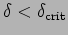) or they spread through the wavenumber space infinitely (if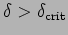), as happens on Figure (1).

 [width=5cm]gen01.eps [width=5cm]gen02.eps [width=5cm]gen03.eps [width=5cm]gen04.eps [width=5cm]gen05.eps [width=5cm]gen06.eps [width=5cm]gen07.eps [width=5cm]gen08.eps [width=5cm]gen09.eps gen01.eps gen02.eps gen03.eps gen04.eps gen05.eps gen06.eps gen07.eps gen08.eps gen09.epsNext: Numerical simulation Up: Discreteness and its effect Previous: Steady state solutions.
Dr Yuri V Lvov 2007-01-16